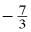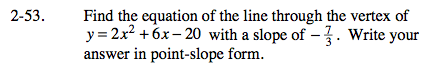### Home > CALC > Chapter 2 > Lesson 2.2.1 > Problem2-53

2-53.

Find the equation of the line through the vertex of y = 2x2 + 6x − 20 with a slope of. Write your answer in point-slope form. Homework Help ✎Find the coordinate of the vertex.

There are many ways to find a vertex. One way is by using the formula:

This will give you the x-value of the vertex. Then evaluate y = 2x2 + 6x − 20 to find the y-coordinate.

Find the equation of the line in point-slope form.

Since the slope is given and a point is known (the vertex), find the equation of the line in point-slope form: yy1 = m(xx1)